Want to share your content on R-bloggers? click here if you have a blog, or here if you don't.

In my last post, I analysed the feature importance for the per cent of engineers in Sweden who are women. I found that the size of the region is a feature that is significant for the per cent of engineers in Sweden who are women. The size of the region is correlated to the number of engineers that works in the region. In this post, I will analyse what predictors are best to forecast the number of engineers in the region. I will use models from the caret package in my analysis.

Statistics Sweden use NUTS (Nomenclature des Unités Territoriales Statistiques), which is the EU’s hierarchical regional division, to specify the regions.

Please send suggestions for improvement of the analysis to .

First, define libraries and functions.

`library (tidyverse)`

`## -- Attaching packages -------------------------------------------------- tidyverse 1.3.0 --`

```## v ggplot2 3.3.0     v purrr   0.3.4
## v tibble  3.0.0     v dplyr   0.8.5
## v tidyr   1.0.2     v stringr 1.4.0
## v readr   1.3.1     v forcats 0.5.0```

```## -- Conflicts ----------------------------------------------------- tidyverse_conflicts() --

```library (broom)
library (car)```

`## Loading required package: carData`

```##
## Attaching package: 'car'```

```## The following object is masked from 'package:dplyr':
##
##     recode```

```## The following object is masked from 'package:purrr':
##
##     some```

`library (caret)`

`## Loading required package: lattice`

```##
## Attaching package: 'caret'```

```## The following object is masked from 'package:purrr':
##
##     lift```

`library (recipes)`

```##
## Attaching package: 'recipes'```

```## The following object is masked from 'package:stringr':
##
##     fixed```

```## The following object is masked from 'package:stats':
##
##     step```

`library (PerformanceAnalytics)`

`## Loading required package: xts`

`## Loading required package: zoo`

```##
## Attaching package: 'zoo'```

```## The following objects are masked from 'package:base':
##
##     as.Date, as.Date.numeric```

```##
## Attaching package: 'xts'```

```## The following objects are masked from 'package:dplyr':
##
##     first, last```

```##
## Attaching package: 'PerformanceAnalytics'```

```## The following object is masked from 'package:graphics':
##
##     legend```

`library (ggpubr)`

`## Loading required package: magrittr`

```##
## Attaching package: 'magrittr'```

```## The following object is masked from 'package:purrr':
##
##     set_names```

```## The following object is masked from 'package:tidyr':
##
##     extract```

```library (ipred)
library (iml)
library (DALEX)```

```## Welcome to DALEX (version: 1.2.1).
## Find examples and detailed introduction at: https://pbiecek.github.io/ema/```

```##
## Attaching package: 'DALEX'```

```## The following object is masked from 'package:dplyr':
##
##     explain```

`library (Metrics)`

```##
## Attaching package: 'Metrics'```

```## The following objects are masked from 'package:caret':
##
##     precision, recall```

`library (auditor)`

```##
## Attaching package: 'auditor'```

```## The following object is masked from 'package:DALEX':
##
##     model_performance```

```readfile <- function (file1){read_csv (file1, col_types = cols(), locale = readr::locale (encoding = "latin1"), na = c("..", "NA")) %>%
gather (starts_with("19"), starts_with("20"), key = "year", value = groupsize) %>%
drop_na() %>%
mutate (year_n = parse_number (year))
}

perc_women <- function(x){
ifelse (length(x) == 2, x / (x + x), NA)
}

mutate(NUTS2_sh = substr(NUTS2, 3, 4))

nuts %>%
distinct (NUTS2_en) %>%
knitr::kable(
booktabs = TRUE,
caption = 'Nomenclature des Unités Territoriales Statistiques (NUTS)')```

Table 1: Nomenclature des Unités Territoriales Statistiques (NUTS)
NUTS2_en
SE11 Stockholm
SE12 East-Central Sweden
SE21 Småland and islands
SE22 South Sweden
SE23 West Sweden
SE31 North-Central Sweden
SE32 Central Norrland
SE33 Upper Norrland

```bs <- function(formula, data, indices) {
d <- data[indices,] # allows boot to select sample
fit <- lm(formula, weights = tbnum_weights, data=d)
return(coef(fit))
}```

The data tables are downloaded from Statistics Sweden. They are saved as a comma-delimited file without heading, UF0506A1.csv, http://www.statistikdatabasen.scb.se/pxweb/en/ssd/.

The tables:

UF0506A1_1.csv: Population 16-74 years of age by region, highest level of education, age and sex. Year 1985 – 2018 NUTS 2 level 2008- 10 year intervals (16-74)

000000CG_1: Average basic salary, monthly salary and women´s salary as a percentage of men´s salary by region, sector, occupational group (SSYK 2012) and sex. Year 2014 – 2018 Monthly salary All sectors.

000000CD_1.csv: Average basic salary, monthly salary and women´s salary as a percentage of men´s salary by region, sector, occupational group (SSYK 2012) and sex. Year 2014 – 2018 Number of employees All sectors-

The data is aggregated, the size of each group is in the column groupsize.

I have also included some calculated predictors from the original data.

perc_women: The percentage of women within each group defined by edulevel, region and year

perc_women_region: The percentage of women within each group defined by year and region

regioneduyears: The average number of education years per capita within each group defined by year and region

eduquotient: The quotient between regioneduyears for men and women

salaryquotient: The quotient between salary for men and women within each group defined by year and region

perc_women_eng_region: The percentage of women who are engineers within each group defined by year and region

```numedulevel <- read.csv("edulevel_1.csv")

numedulevel[, 2] <- data.frame(c(8, 9, 10, 12, 13, 15, 22, NA))

left_join(tb, by = c("region", "year", "sex", "sector","occuptional  (SSYK 2012)")) %>%
filter(`occuptional  (SSYK 2012)` == "214 Engineering professionals")

right_join(tb, by = c("region", "year", "sex")) %>%
right_join(numedulevel, by = c("level of education" = "level.of.education")) %>%
filter(!is.na(eduyears)) %>%
mutate(edulevel = `level of education`) %>%
group_by(edulevel, region, year, sex) %>%
mutate(groupsize_all_ages = sum(groupsize)) %>%
group_by(edulevel, region, year) %>%
mutate (perc_women = perc_women (groupsize_all_ages[1:2])) %>%
mutate (suming = sum(groupsize.x)) %>%
mutate (salary = (groupsize.y * groupsize.x + groupsize.y * groupsize.x)/(groupsize.x + groupsize.x)) %>%
group_by (sex, year, region) %>%
mutate(regioneduyears_sex = sum(groupsize * eduyears) / sum(groupsize)) %>%
mutate(regiongroupsize = sum(groupsize)) %>%
mutate(suming_sex = groupsize.x) %>%
group_by(region, year) %>%
mutate (sum_pop = sum(groupsize)) %>%
mutate (regioneduyears = sum(groupsize * eduyears) / sum(groupsize)) %>%
mutate (perc_women_region = perc_women (regiongroupsize[1:2])) %>%
mutate (eduquotient = regioneduyears_sex / regioneduyears_sex) %>%
mutate (salary_sex = groupsize.y) %>%
mutate (salaryquotient = salary_sex / salary_sex) %>%
mutate (perc_women_eng_region = perc_women(suming_sex[1:2])) %>%
left_join(nuts %>% distinct (NUTS2_en, NUTS2_sh), by = c("region" = "NUTS2_en")) %>%
drop_na()

summary(tb)```

```##     region              age            level of education     sex
##  Length:532         Length:532         Length:532         Length:532
##  Class :character   Class :character   Class :character   Class :character
##  Mode  :character   Mode  :character   Mode  :character   Mode  :character
##
##
##
##      year             groupsize          year_n        sector
##  Length:532         Min.   :   405   Min.   :2014   Length:532
##  Class :character   1st Qu.: 20996   1st Qu.:2015   Class :character
##  Mode  :character   Median : 43656   Median :2016   Mode  :character
##                     Mean   : 64760   Mean   :2016
##                     3rd Qu.:102394   3rd Qu.:2017
##                     Max.   :271889   Max.   :2018
##  occuptional  (SSYK 2012)  groupsize.x       year_n.x     groupsize.y
##  Length:532               Min.   :  340   Min.   :2014   Min.   :34700
##  Class :character         1st Qu.: 1700   1st Qu.:2015   1st Qu.:40300
##  Mode  :character         Median : 3000   Median :2016   Median :42000
##                           Mean   : 5850   Mean   :2016   Mean   :42078
##                           3rd Qu.: 7475   3rd Qu.:2017   3rd Qu.:43925
##                           Max.   :21400   Max.   :2018   Max.   :49400
##     year_n.y       eduyears       edulevel         groupsize_all_ages
##  Min.   :2014   Min.   : 8.00   Length:532         Min.   :   405
##  1st Qu.:2015   1st Qu.: 9.00   Class :character   1st Qu.: 20996
##  Median :2016   Median :12.00   Mode  :character   Median : 43656
##  Mean   :2016   Mean   :12.71                      Mean   : 64760
##  3rd Qu.:2017   3rd Qu.:15.00                      3rd Qu.:102394
##  Max.   :2018   Max.   :22.00                      Max.   :271889
##    perc_women         suming          salary      regioneduyears_sex
##  Min.   :0.3575   Min.   : 2040   Min.   :38376   Min.   :11.18
##  1st Qu.:0.4338   1st Qu.: 3080   1st Qu.:40916   1st Qu.:11.61
##  Median :0.4631   Median : 8950   Median :42866   Median :11.74
##  Mean   :0.4771   Mean   :11701   Mean   :42776   Mean   :11.79
##  3rd Qu.:0.5132   3rd Qu.:20100   3rd Qu.:44444   3rd Qu.:12.04
##  Max.   :0.6423   Max.   :29500   Max.   :48429   Max.   :12.55
##  regiongroupsize    suming_sex       sum_pop        regioneduyears
##  Min.   :128262   Min.   :  340   Min.   : 262870   Min.   :11.39
##  1st Qu.:288058   1st Qu.: 1700   1st Qu.: 587142   1st Qu.:11.54
##  Median :514608   Median : 3000   Median :1029820   Median :11.81
##  Mean   :453318   Mean   : 5850   Mean   : 906635   Mean   :11.79
##  3rd Qu.:691870   3rd Qu.: 7475   3rd Qu.:1395157   3rd Qu.:11.90
##  Max.   :827940   Max.   :21400   Max.   :1655215   Max.   :12.41
##  perc_women_region  eduquotient      salary_sex    salaryquotient
##  Min.   :0.4831    Min.   :1.019   Min.   :34700   Min.   :0.8653
##  1st Qu.:0.4882    1st Qu.:1.029   1st Qu.:40300   1st Qu.:0.9329
##  Median :0.4934    Median :1.034   Median :42000   Median :0.9395
##  Mean   :0.4923    Mean   :1.034   Mean   :42078   Mean   :0.9447
##  3rd Qu.:0.4970    3rd Qu.:1.041   3rd Qu.:43925   3rd Qu.:0.9537
##  Max.   :0.5014    Max.   :1.047   Max.   :49400   Max.   :1.0446
##  perc_women_eng_region   NUTS2_sh
##  Min.   :0.1566        Length:532
##  1st Qu.:0.1787        Class :character
##  Median :0.2042        Mode  :character
##  Mean   :0.2039
##  3rd Qu.:0.2216
##  Max.   :0.2746```

Prepare the data using Tidyverse recipes package, i.e. centre, scale and make sure all predictors are numerical.

```tbtemp <- ungroup(tb) %>% dplyr::select(region, salary, year_n, sum_pop, regioneduyears, suming, perc_women_region, salaryquotient, eduquotient, perc_women_eng_region)

tb_outliers_info <- unique(tbtemp)

tb_unique <- unique(dplyr::select(tbtemp, -region))

tbnum_weights <- tb_unique\$suming

blueprint <- recipe(suming ~ ., data = tb_unique) %>%
step_integer(matches("Qual|Cond|QC|Qu")) %>%
step_center(all_numeric(), -all_outcomes()) %>%
step_scale(all_numeric(), -all_outcomes()) %>%
step_dummy(all_nominal(), -all_outcomes(), one_hot = TRUE)

prepare <- prep(blueprint, training = tb_unique)

tbnum <- bake(prepare, new_data = tb_unique)```

The correlation chart shows that many predictors are correlated with the response variable but also that many predictors are correlated between each other. Some notable correlations are in a dedicated plot below.

`chart.Correlation(tbnum, histogram = TRUE, pch = 19)`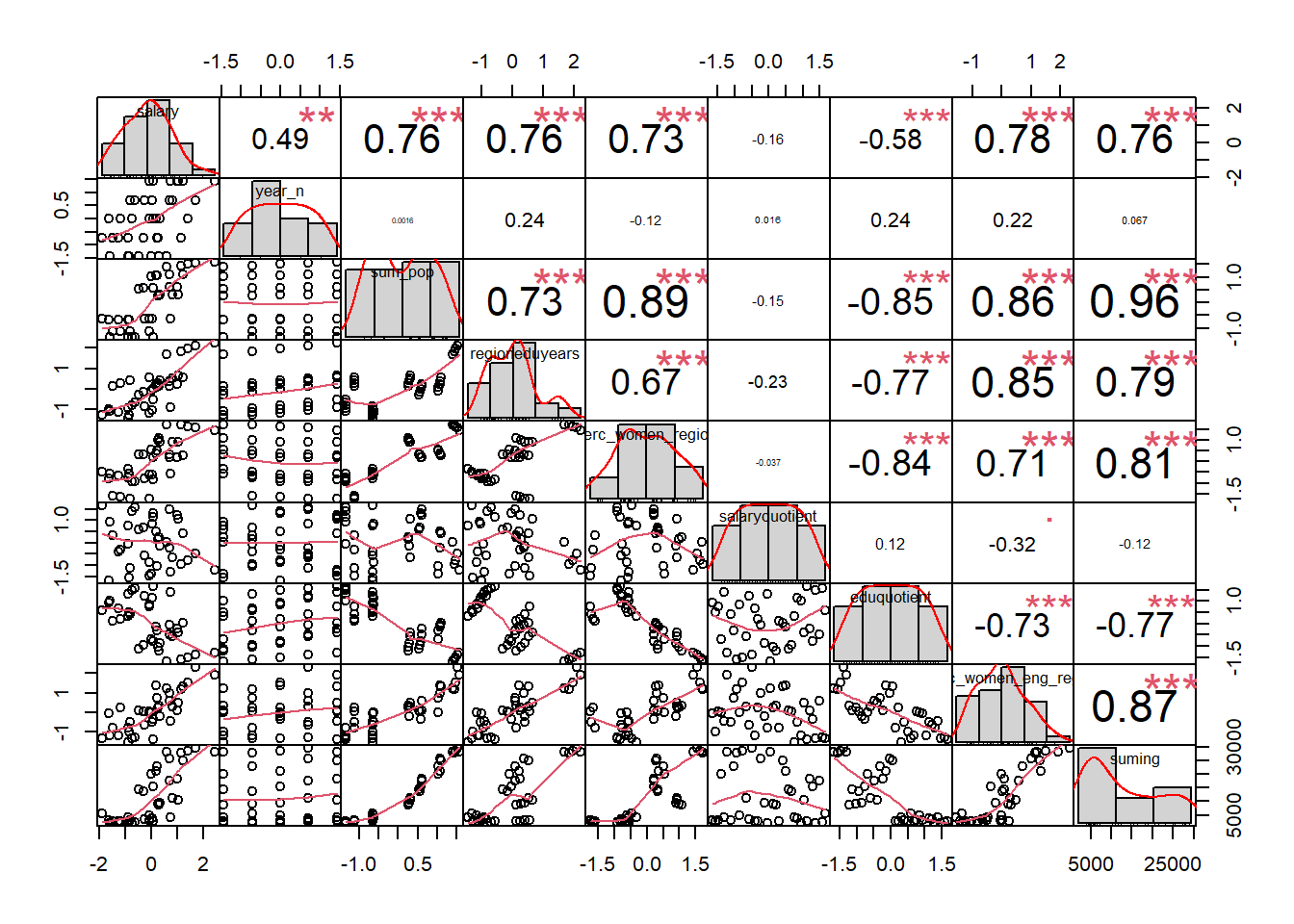Figure 1: Correlation between response and predictors and between predictors, Year 2014 – 2018

```p1 <- tb %>%
ggscatter(x = "sum_pop", y = "suming",
add = "reg.line", conf.int = TRUE,
cor.coef = TRUE, cor.method = "pearson")

p2 <- tb %>%
ggscatter(x = "sum_pop", y = "perc_women_region",
add = "reg.line", conf.int = TRUE,
cor.coef = TRUE, cor.method = "pearson")

p3 <- tb %>%
ggscatter(x = "suming", y = "perc_women_eng_region",
add = "reg.line", conf.int = TRUE,
cor.coef = TRUE, cor.method = "pearson")

p4 <- tb %>%
ggscatter(x = "sum_pop", y = "perc_women_eng_region",
add = "reg.line", conf.int = TRUE,
cor.coef = TRUE, cor.method = "pearson")

gridExtra::grid.arrange(p1, p2, p3, p4, ncol = 2)```

```## `geom_smooth()` using formula 'y ~ x'
## `geom_smooth()` using formula 'y ~ x'
## `geom_smooth()` using formula 'y ~ x'
## `geom_smooth()` using formula 'y ~ x'```Figure 2: Correlation between response and predictors and between predictors, Year 2014 – 2018

plot_model will show diagnostics for the model, a residual plot, scale location plot, residual density and the regression error characteristic curve. It will also show the residuals versus the actual response to help find outliers. It will plot the feature importance and feature effects. In addition, it will plot how strongly features interact with each other and the 2-way interactions between the feature with the strongest interaction and all other features. The interaction measure regards how much of the variance of f(x) is explained by the interaction. The measure is between 0 (no interaction) and 1 (= 100% of variance of f(x) due to interactions).

```plot_model <- function(model){
invisible(capture.output(exp_model <- DALEX::explain(model, data = tbnum, y = tbnum\$suming))) # Knit and DALEX::explain generates invalid rss feed

lm_mr <- model_residual(exp_model)

predictor <- Predictor\$new(model,
data = dplyr::select(tbnum, -suming),
y = tbnum\$suming)

writeLines("")

print(model)

print(postResample(pred = predict(model), obs = tbnum\$suming))

p1 <- plot(lm_mr, type = "residual")

p2 <- plot(lm_mr, type = "scalelocation")

p3 <- plot(lm_mr, type = "residual_density")

p4 <- plot(lm_mr, type = "rec")

print(gridExtra::grid.arrange(p1, p2, p3, p4, ncol = 2))

print(plot_residual(lm_mr, variable = "_y_hat_", nlabel = 10))

print(plot (FeatureImp\$new(predictor, loss = "mae")))

print(plot (FeatureEffects\$new(predictor)))

interact <- Interaction\$new(predictor)

p1 <- plot (interact)

p2 <- plot (Interaction\$new(predictor, feature = as.character(arrange(interact\$results, desc(.interaction))[1,1])))

print(gridExtra::grid.arrange(p1, p2, ncol = 2))
}```

Fit the following models and plot inference and diagnostics. Principal component analysis (PCA) is used to transform the data into a smaller subspace where the new variables are uncorrelated with one another due to the high multicollinearity.
Linear Regression, Projection Pursuit Regression, Bagged MARS, Random Forest, Bagged CART, Boosted Tree, Conditional Inference Tree

```modelcollection <- c("lm", "ppr", "bagEarth", "ranger", "treebag", "blackboost", "ctree")

for (model in modelcollection){
invisible(capture.output(model <- caret::train(
suming ~ .,
data = tbnum,
method = model,
preProc=c("pca"),
weights = tbnum_weights,
trControl = trainControl(method = "cv", number = 10)
)))

plot_model(model)
}```

```##
## Linear Regression
##
## 38 samples
##  8 predictor
##
## Pre-processing: principal component signal extraction (8), centered (8),
##  scaled (8)
## Resampling: Cross-Validated (10 fold)
## Summary of sample sizes: 35, 34, 34, 35, 34, 34, ...
## Resampling results:
##
##   RMSE      Rsquared   MAE
##   4237.874  0.8822043  3423.46
##
## Tuning parameter 'intercept' was held constant at a value of TRUE
##         RMSE     Rsquared          MAE
## 3883.8558386    0.8463367 2767.1742230```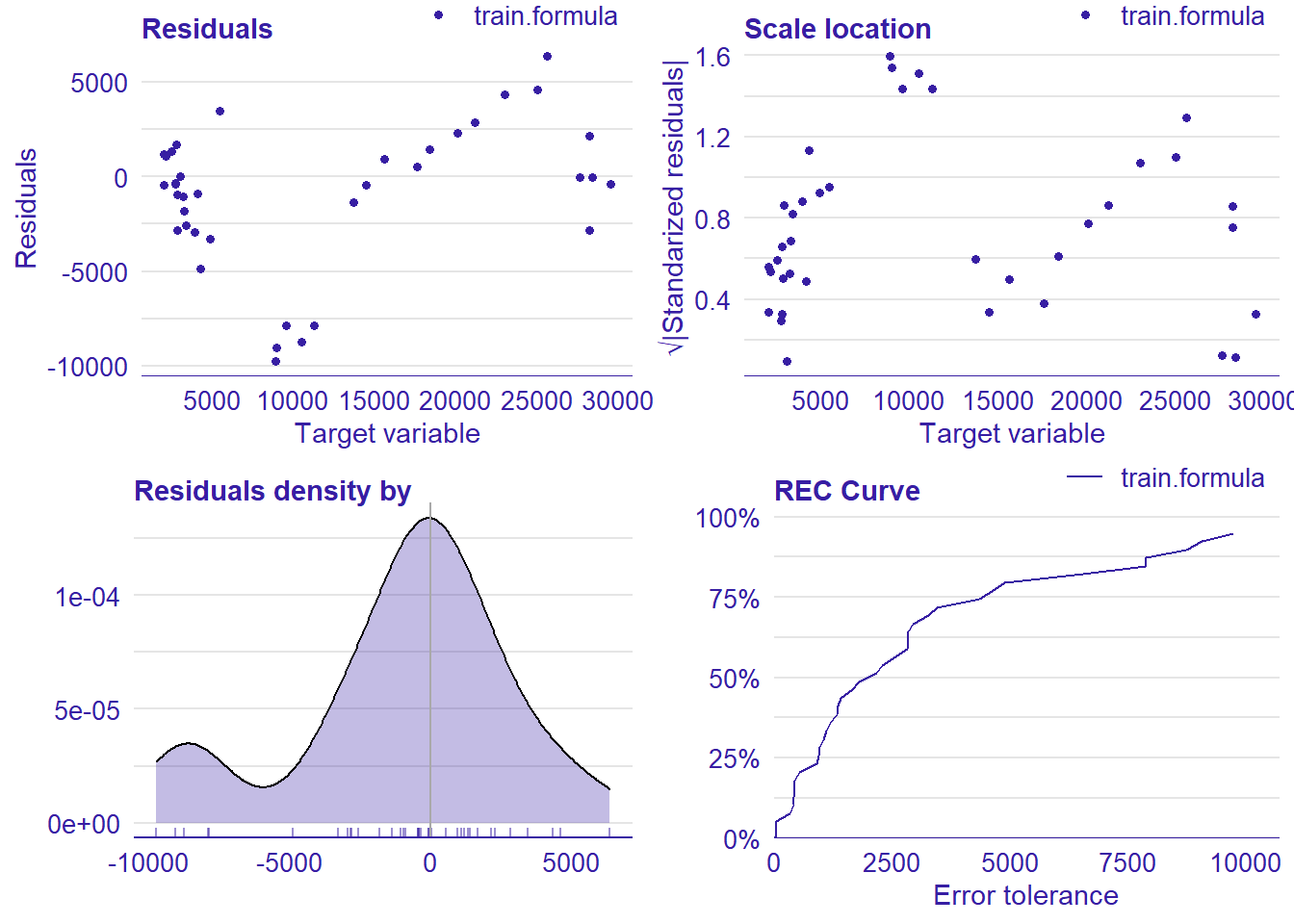Figure 3: , Year 2014 – 2018

```## TableGrob (2 x 2) "arrange": 4 grobs
##   z     cells    name           grob
## 1 1 (1-1,1-1) arrange gtable[layout]
## 2 2 (1-1,2-2) arrange gtable[layout]
## 3 3 (2-2,1-1) arrange gtable[layout]
## 4 4 (2-2,2-2) arrange gtable[layout]```Figure 4: , Year 2014 – 2018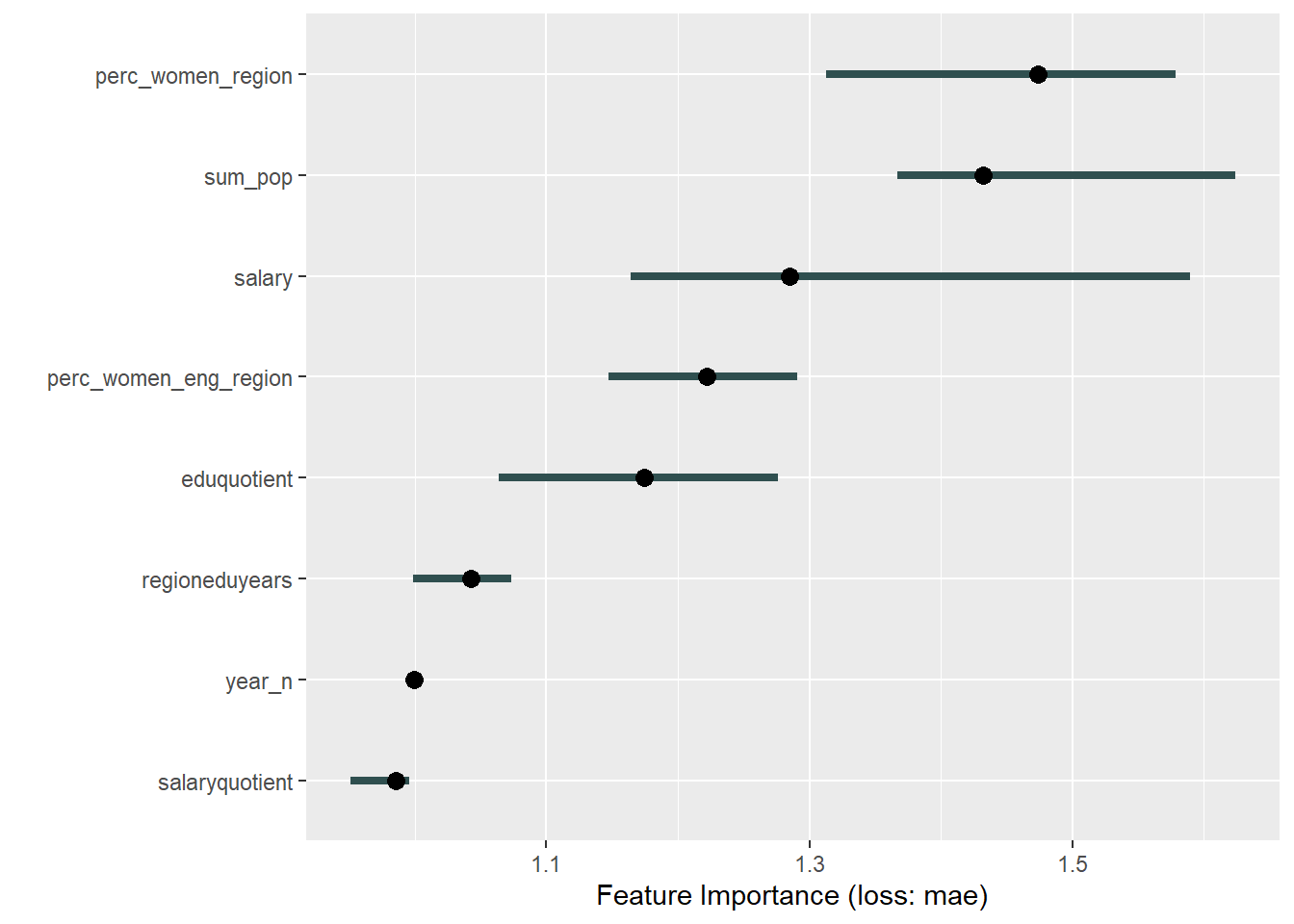Figure 5: , Year 2014 – 2018

```##
## Attaching package: 'patchwork'```

```## The following object is masked from 'package:MASS':
##
##     area```Figure 6: , Year 2014 – 2018Figure 7: , Year 2014 – 2018

```## TableGrob (1 x 2) "arrange": 2 grobs
##   z     cells    name           grob
## 1 1 (1-1,1-1) arrange gtable[layout]
## 2 2 (1-1,2-2) arrange gtable[layout]
##
## Projection Pursuit Regression
##
## 38 samples
##  8 predictor
##
## Pre-processing: principal component signal extraction (8), centered (8),
##  scaled (8)
## Resampling: Cross-Validated (10 fold)
## Summary of sample sizes: 34, 34, 34, 34, 34, 34, ...
## Resampling results across tuning parameters:
##
##   nterms  RMSE      Rsquared   MAE
##   1       4130.062  0.8121472  3256.277
##   2       4246.374  0.8418553  3408.002
##   3       3906.203  0.8768507  3171.871
##
## RMSE was used to select the optimal model using the smallest value.
## The final value used for the model was nterms = 3.
##         RMSE     Rsquared          MAE
## 1402.1162486    0.9796416 1090.5426055```Figure 8: , Year 2014 – 2018

```## TableGrob (2 x 2) "arrange": 4 grobs
##   z     cells    name           grob
## 1 1 (1-1,1-1) arrange gtable[layout]
## 2 2 (1-1,2-2) arrange gtable[layout]
## 3 3 (2-2,1-1) arrange gtable[layout]
## 4 4 (2-2,2-2) arrange gtable[layout]```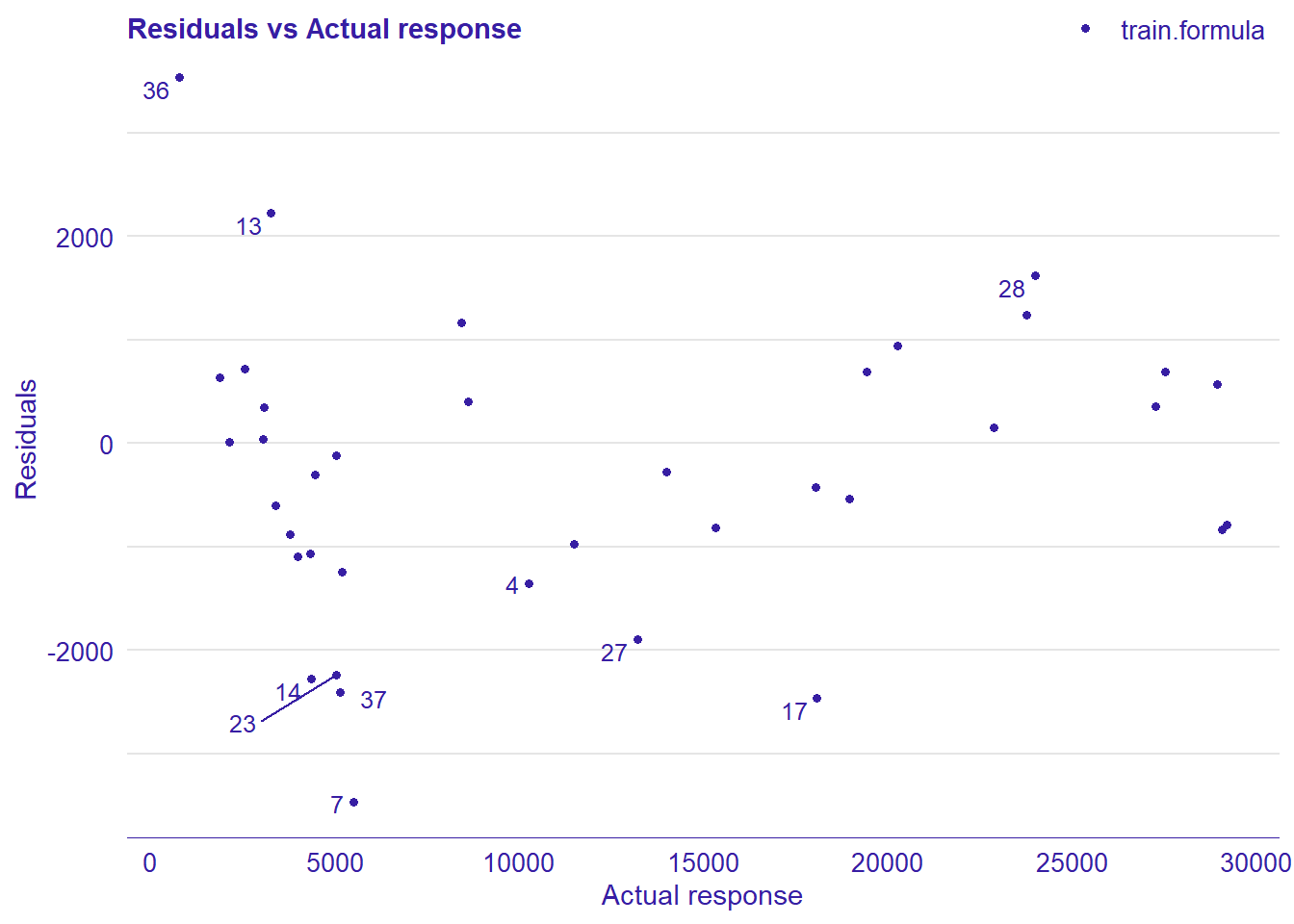Figure 9: , Year 2014 – 2018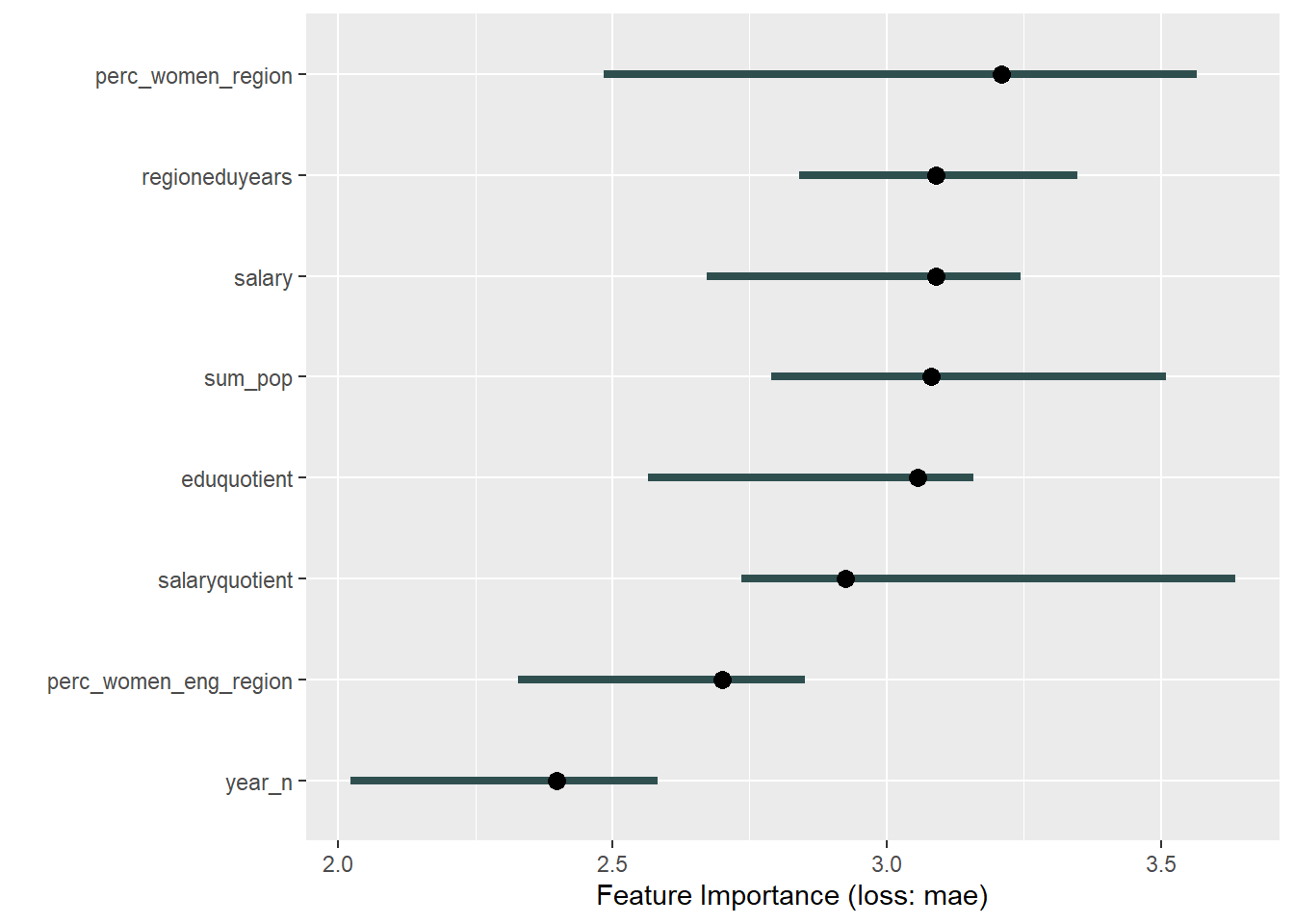Figure 10: , Year 2014 – 2018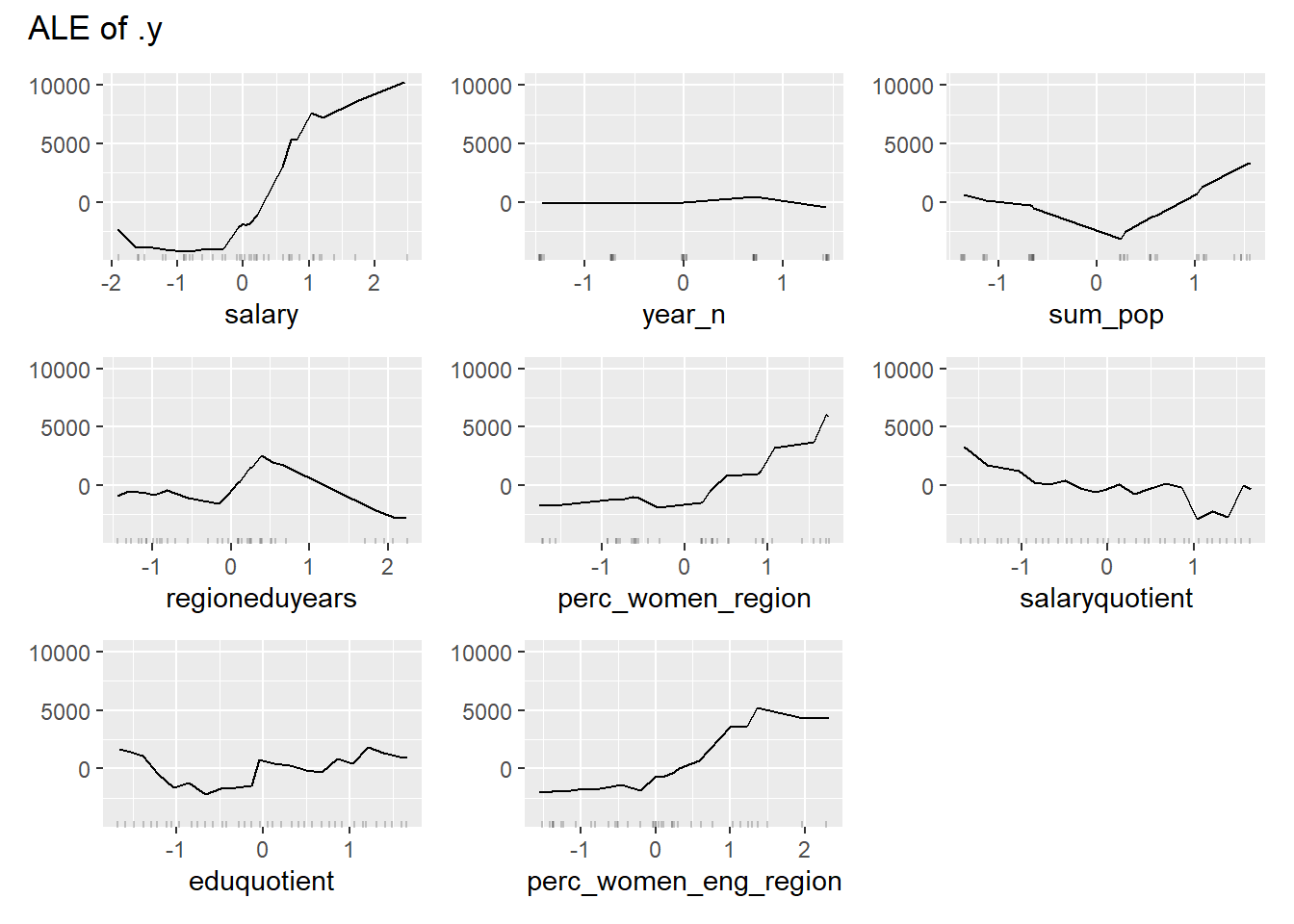Figure 11: , Year 2014 – 2018

```## TableGrob (1 x 2) "arrange": 2 grobs
##   z     cells    name           grob
## 1 1 (1-1,1-1) arrange gtable[layout]
## 2 2 (1-1,2-2) arrange gtable[layout]```

`## Loading required package: earth`

`## Loading required package: Formula`

`## Loading required package: plotmo`

`## Loading required package: plotrix`

```##
## Attaching package: 'plotrix'```

```## The following object is masked from 'package:scales':
##
##     rescale```

`## Loading required package: TeachingDemos`Figure 12: , Year 2014 – 2018

```##
## Bagged MARS
##
## 38 samples
##  8 predictor
##
## Pre-processing: principal component signal extraction (8), centered (8),
##  scaled (8)
## Resampling: Cross-Validated (10 fold)
## Summary of sample sizes: 34, 34, 34, 34, 35, 34, ...
## Resampling results across tuning parameters:
##
##   nprune  RMSE      Rsquared   MAE
##    2      4671.353  0.8678650  3762.492
##    7      4301.170  0.8466750  3379.813
##   13      4535.948  0.8434574  3713.592
##
## Tuning parameter 'degree' was held constant at a value of 1
## RMSE was used to select the optimal model using the smallest value.
## The final values used for the model were nprune = 7 and degree = 1.
##         RMSE     Rsquared          MAE
## 2950.1269148    0.9075753 2178.7339222```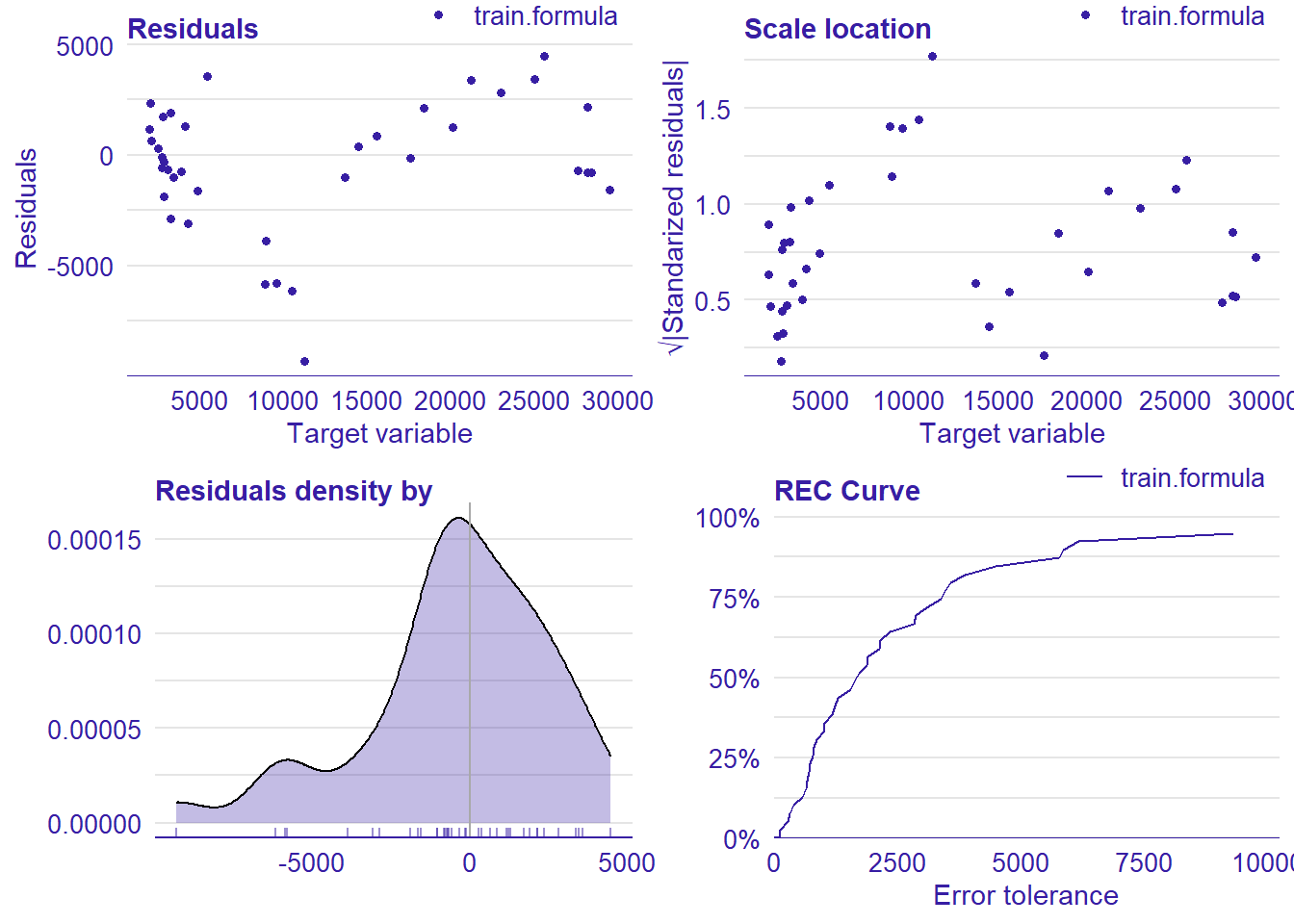Figure 13: , Year 2014 – 2018

```## TableGrob (2 x 2) "arrange": 4 grobs
##   z     cells    name           grob
## 1 1 (1-1,1-1) arrange gtable[layout]
## 2 2 (1-1,2-2) arrange gtable[layout]
## 3 3 (2-2,1-1) arrange gtable[layout]
## 4 4 (2-2,2-2) arrange gtable[layout]```Figure 14: , Year 2014 – 2018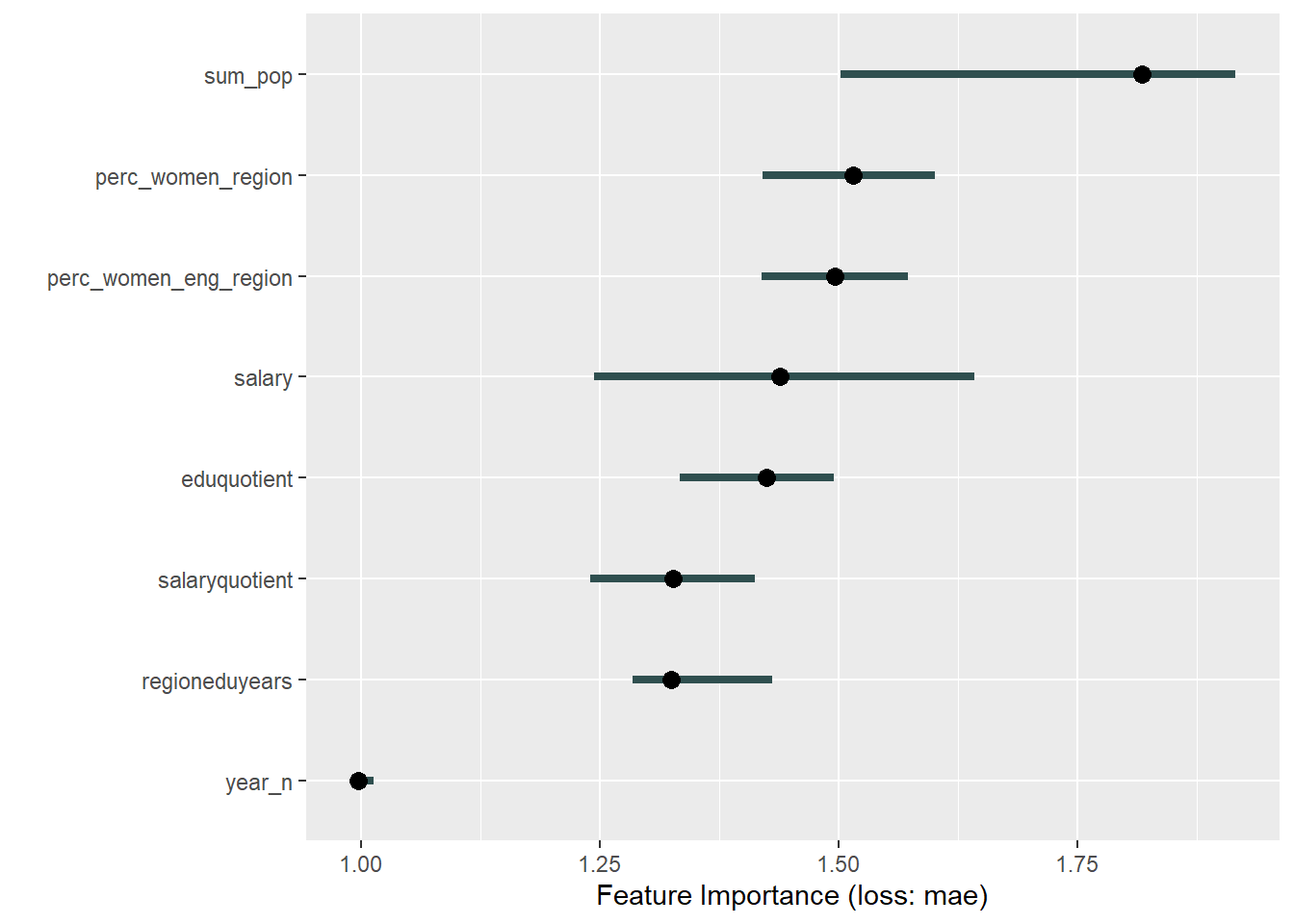Figure 15: , Year 2014 – 2018Figure 16: , Year 2014 – 2018Figure 17: , Year 2014 – 2018

```## TableGrob (1 x 2) "arrange": 2 grobs
##   z     cells    name           grob
## 1 1 (1-1,1-1) arrange gtable[layout]
## 2 2 (1-1,2-2) arrange gtable[layout]
##
## Random Forest
##
## 38 samples
##  8 predictor
##
## Pre-processing: principal component signal extraction (8), centered (8),
##  scaled (8)
## Resampling: Cross-Validated (10 fold)
## Summary of sample sizes: 34, 34, 34, 34, 34, 34, ...
## Resampling results across tuning parameters:
##
##   mtry  splitrule   RMSE      Rsquared   MAE
##   2     variance    6106.457  0.7899054  5600.253
##   2     extratrees  5899.990  0.8178129  5413.206
##   3     variance    5242.999  0.8372295  4583.812
##   3     extratrees  4978.308  0.8145967  4339.194
##   4     variance    4367.341  0.8690214  3526.925
##   4     extratrees  4461.317  0.8391514  3797.024
##
## Tuning parameter 'min.node.size' was held constant at a value of 5
## RMSE was used to select the optimal model using the smallest value.
## The final values used for the model were mtry = 4, splitrule = variance
##  and min.node.size = 5.
##         RMSE     Rsquared          MAE
## 1782.3243833    0.9749664 1275.2575351```Figure 18: , Year 2014 – 2018

```## TableGrob (2 x 2) "arrange": 4 grobs
##   z     cells    name           grob
## 1 1 (1-1,1-1) arrange gtable[layout]
## 2 2 (1-1,2-2) arrange gtable[layout]
## 3 3 (2-2,1-1) arrange gtable[layout]
## 4 4 (2-2,2-2) arrange gtable[layout]```Figure 19: , Year 2014 – 2018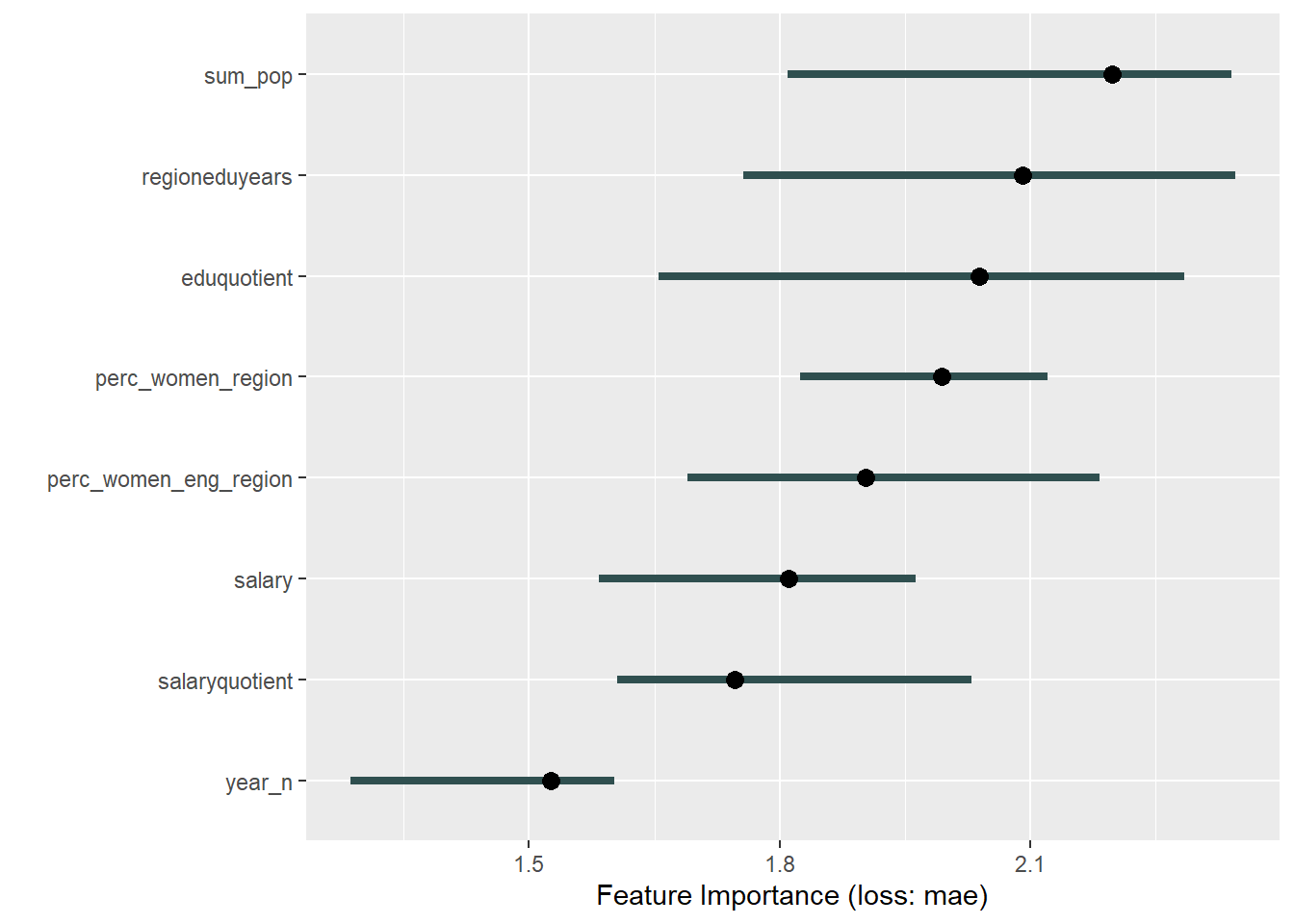Figure 20: , Year 2014 – 2018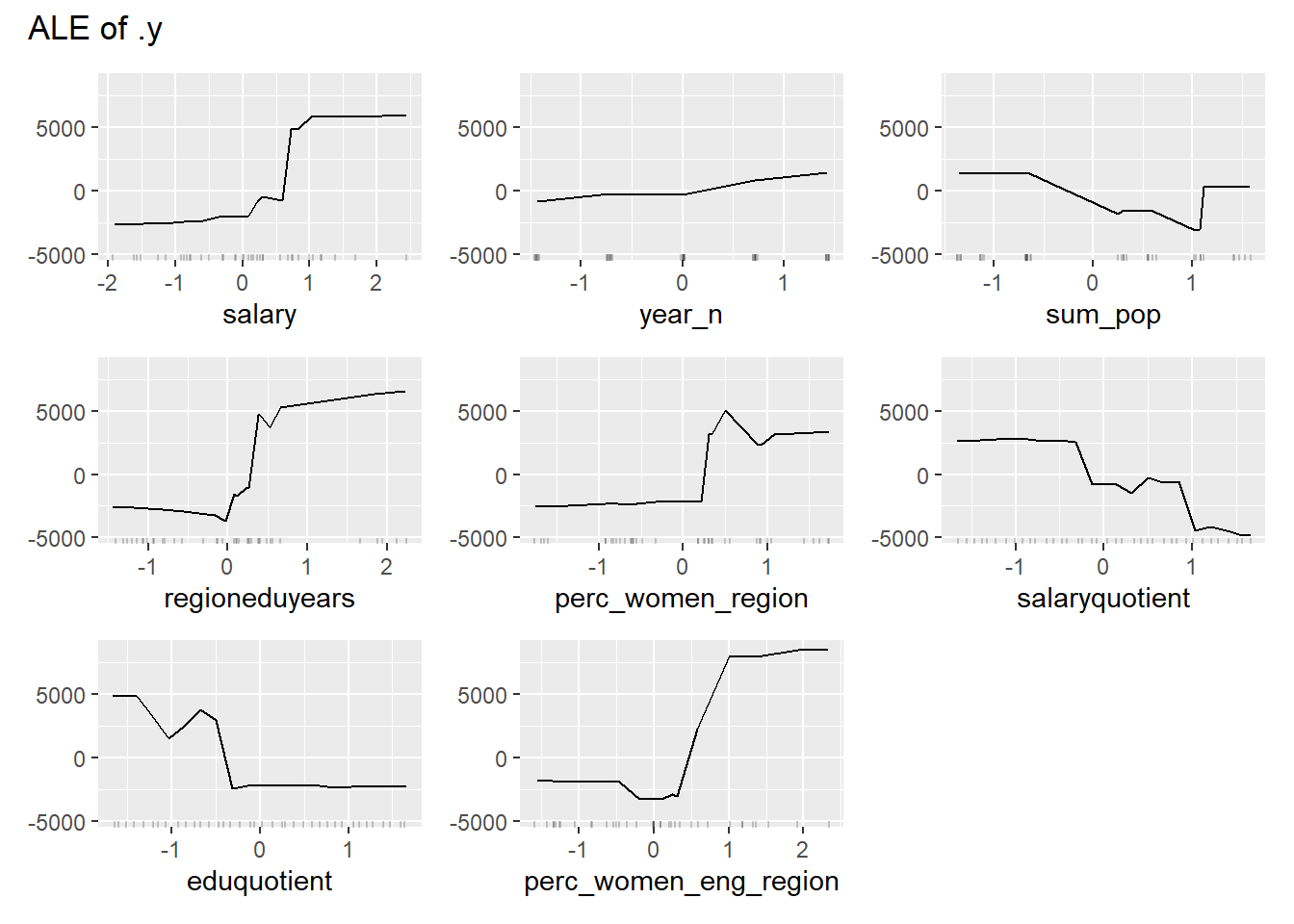Figure 21: , Year 2014 – 2018Figure 22: , Year 2014 – 2018

```## TableGrob (1 x 2) "arrange": 2 grobs
##   z     cells    name           grob
## 1 1 (1-1,1-1) arrange gtable[layout]
## 2 2 (1-1,2-2) arrange gtable[layout]
##
## Bagged CART
##
## 38 samples
##  8 predictor
##
## Pre-processing: principal component signal extraction (8), centered (8),
##  scaled (8)
## Resampling: Cross-Validated (10 fold)
## Summary of sample sizes: 35, 34, 34, 34, 34, 34, ...
## Resampling results:
##
##   RMSE      Rsquared   MAE
##   4828.894  0.8094027  3527.702
##
##         RMSE     Rsquared          MAE
## 3481.9118680    0.8750994 2582.9979981```Figure 23: , Year 2014 – 2018

```## TableGrob (2 x 2) "arrange": 4 grobs
##   z     cells    name           grob
## 1 1 (1-1,1-1) arrange gtable[layout]
## 2 2 (1-1,2-2) arrange gtable[layout]
## 3 3 (2-2,1-1) arrange gtable[layout]
## 4 4 (2-2,2-2) arrange gtable[layout]```Figure 24: , Year 2014 – 2018Figure 25: , Year 2014 – 2018Figure 26: , Year 2014 – 2018

```## TableGrob (1 x 2) "arrange": 2 grobs
##   z     cells    name           grob
## 1 1 (1-1,1-1) arrange gtable[layout]
## 2 2 (1-1,2-2) arrange gtable[layout]```

```## Warning in if (class(response) == "data.frame") response <- as.matrix(response):
## the condition has length > 1 and only the first element will be used

## Warning in if (class(response) == "data.frame") response <- as.matrix(response):
## the condition has length > 1 and only the first element will be used```Figure 27: , Year 2014 – 2018

```##
## Boosted Tree
##
## 38 samples
##  8 predictor
##
## Pre-processing: principal component signal extraction (8), centered (8),
##  scaled (8)
## Resampling: Cross-Validated (10 fold)
## Summary of sample sizes: 34, 34, 34, 34, 34, 35, ...
## Resampling results across tuning parameters:
##
##   maxdepth  mstop  RMSE      Rsquared   MAE
##   1          50    4440.664  0.7708467  3558.175
##   1         100    4429.005  0.7943325  3512.541
##   1         150    4456.381  0.7981314  3487.719
##   2          50    4598.712  0.8028983  3497.232
##   2         100    4582.388  0.7786909  3482.167
##   2         150    4546.031  0.7990669  3437.192
##   3          50    4166.522  0.8926944  2940.884
##   3         100    4134.064  0.8935203  2910.923
##   3         150    4139.154  0.8939033  2918.382
##
## RMSE was used to select the optimal model using the smallest value.
## The final values used for the model were mstop = 100 and maxdepth = 3.
##        RMSE    Rsquared         MAE
## 110.7432126   0.9998769  88.9875261```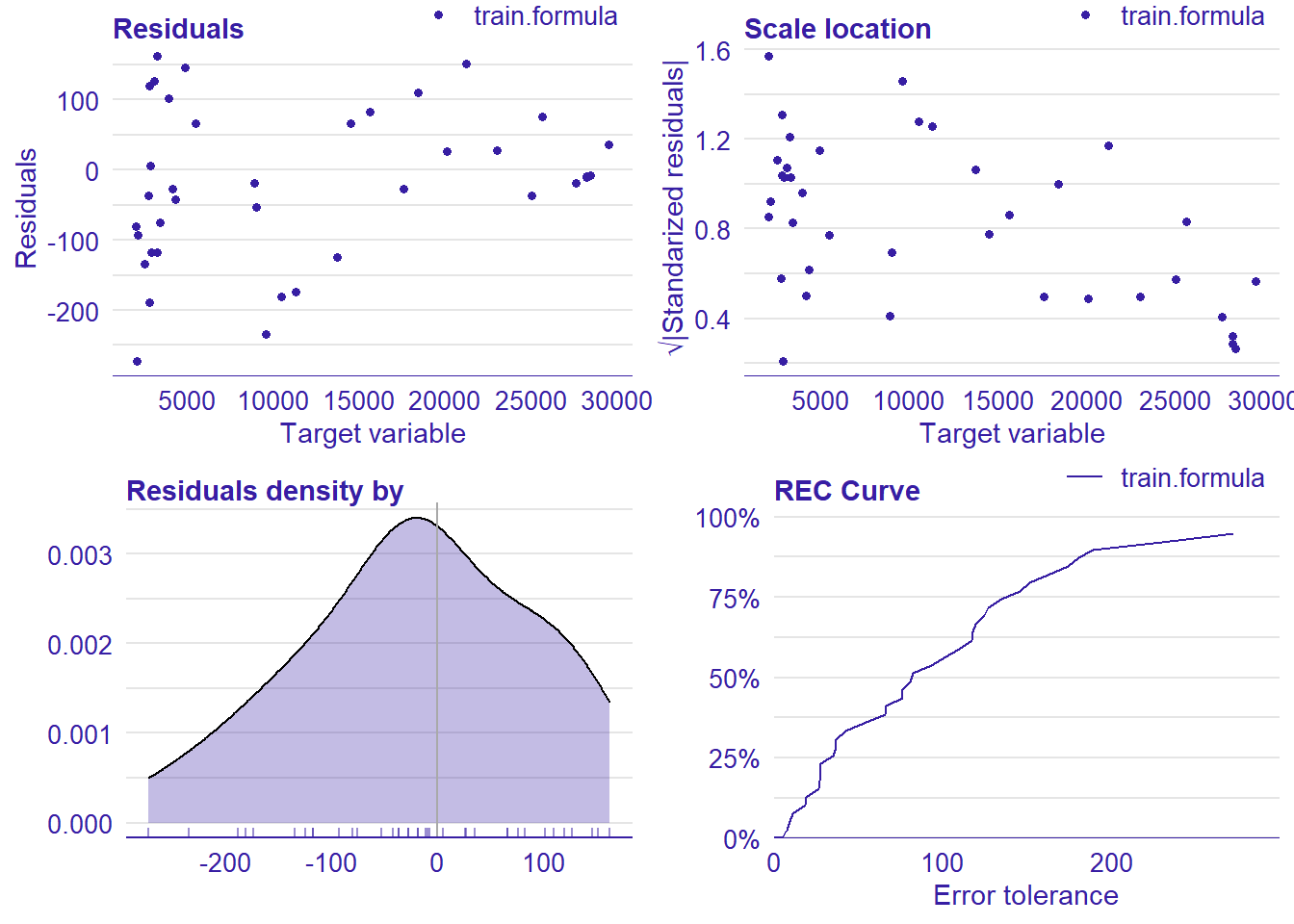Figure 28: , Year 2014 – 2018

```## TableGrob (2 x 2) "arrange": 4 grobs
##   z     cells    name           grob
## 1 1 (1-1,1-1) arrange gtable[layout]
## 2 2 (1-1,2-2) arrange gtable[layout]
## 3 3 (2-2,1-1) arrange gtable[layout]
## 4 4 (2-2,2-2) arrange gtable[layout]```Figure 29: , Year 2014 – 2018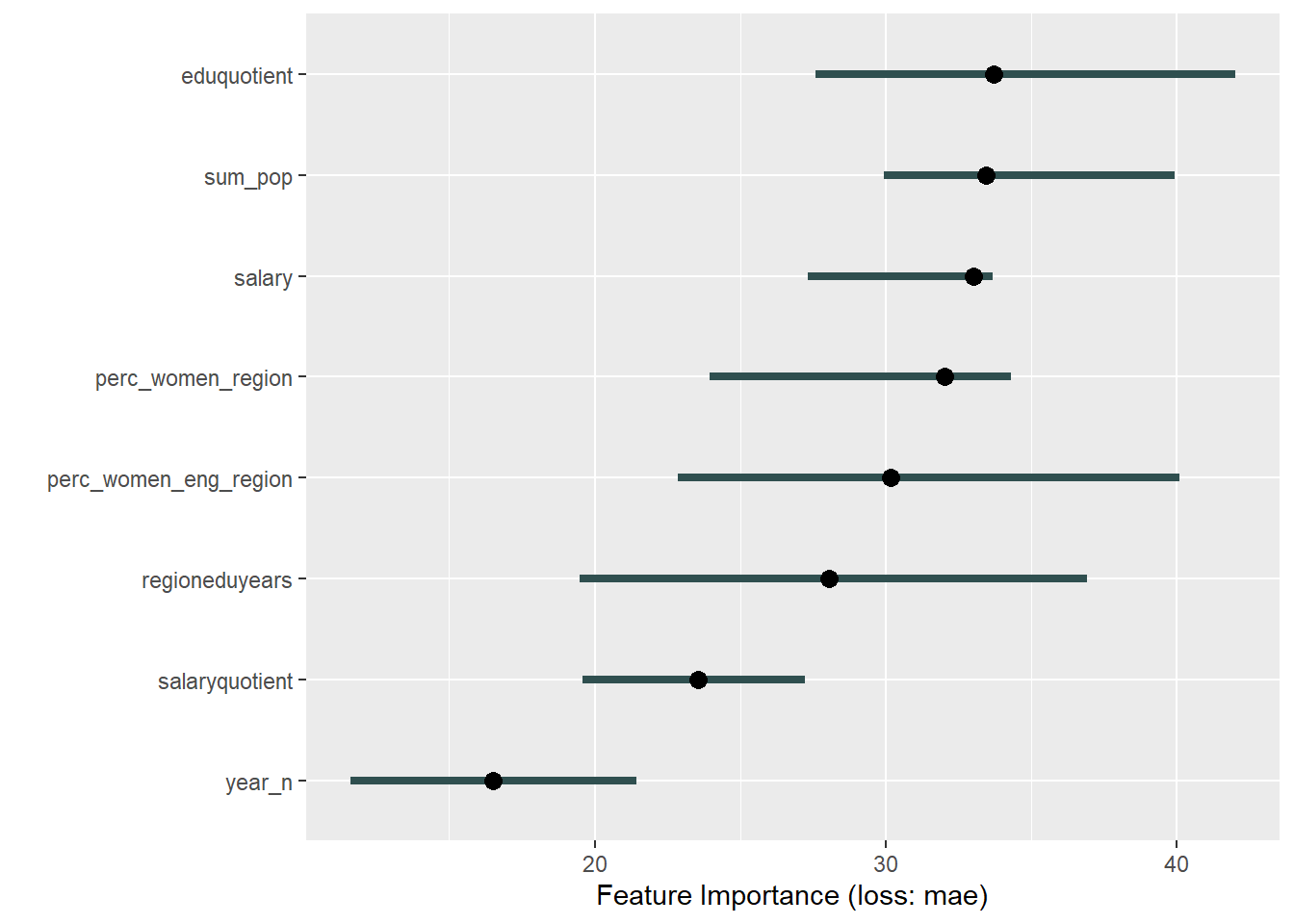Figure 30: , Year 2014 – 2018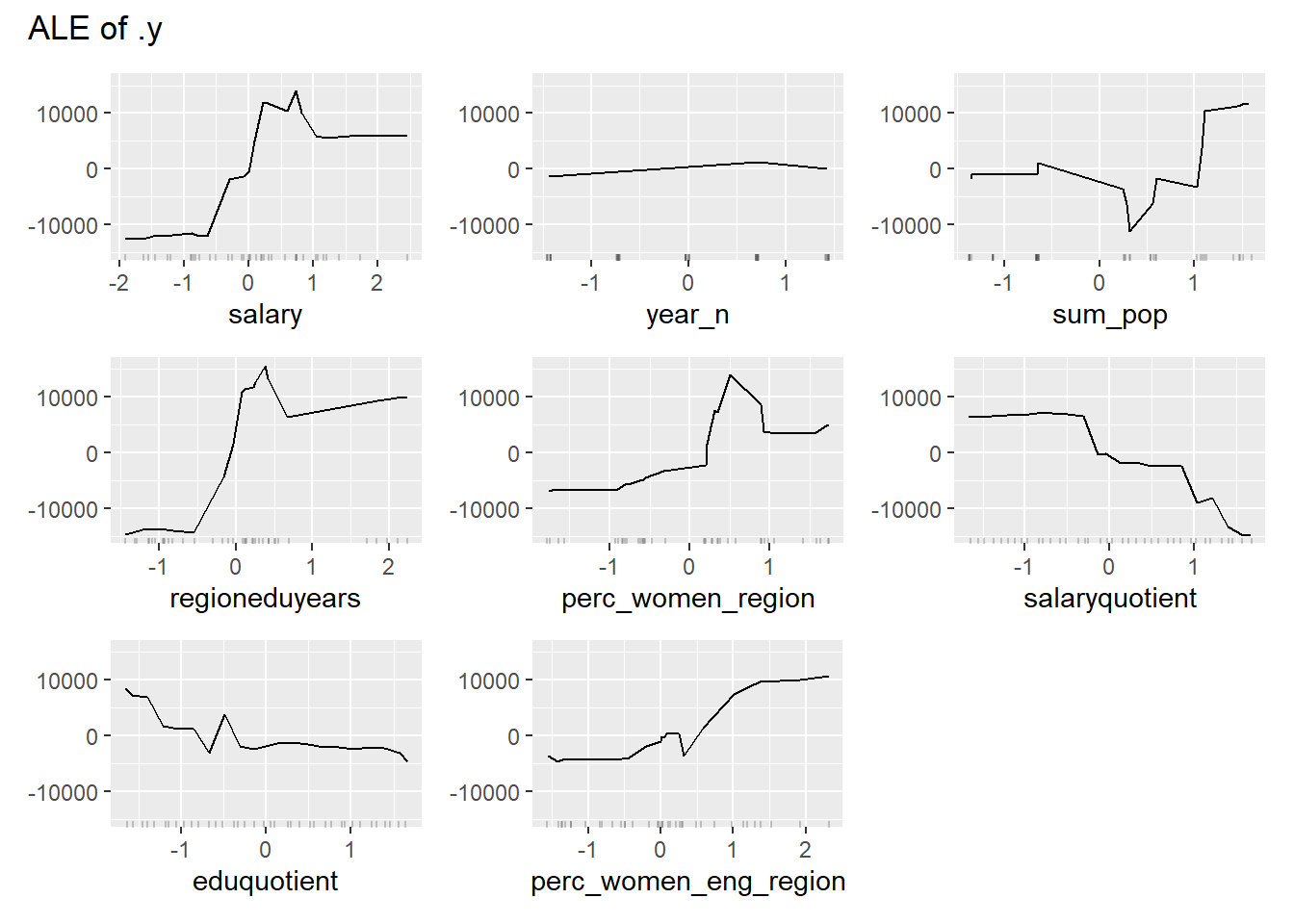Figure 31: , Year 2014 – 2018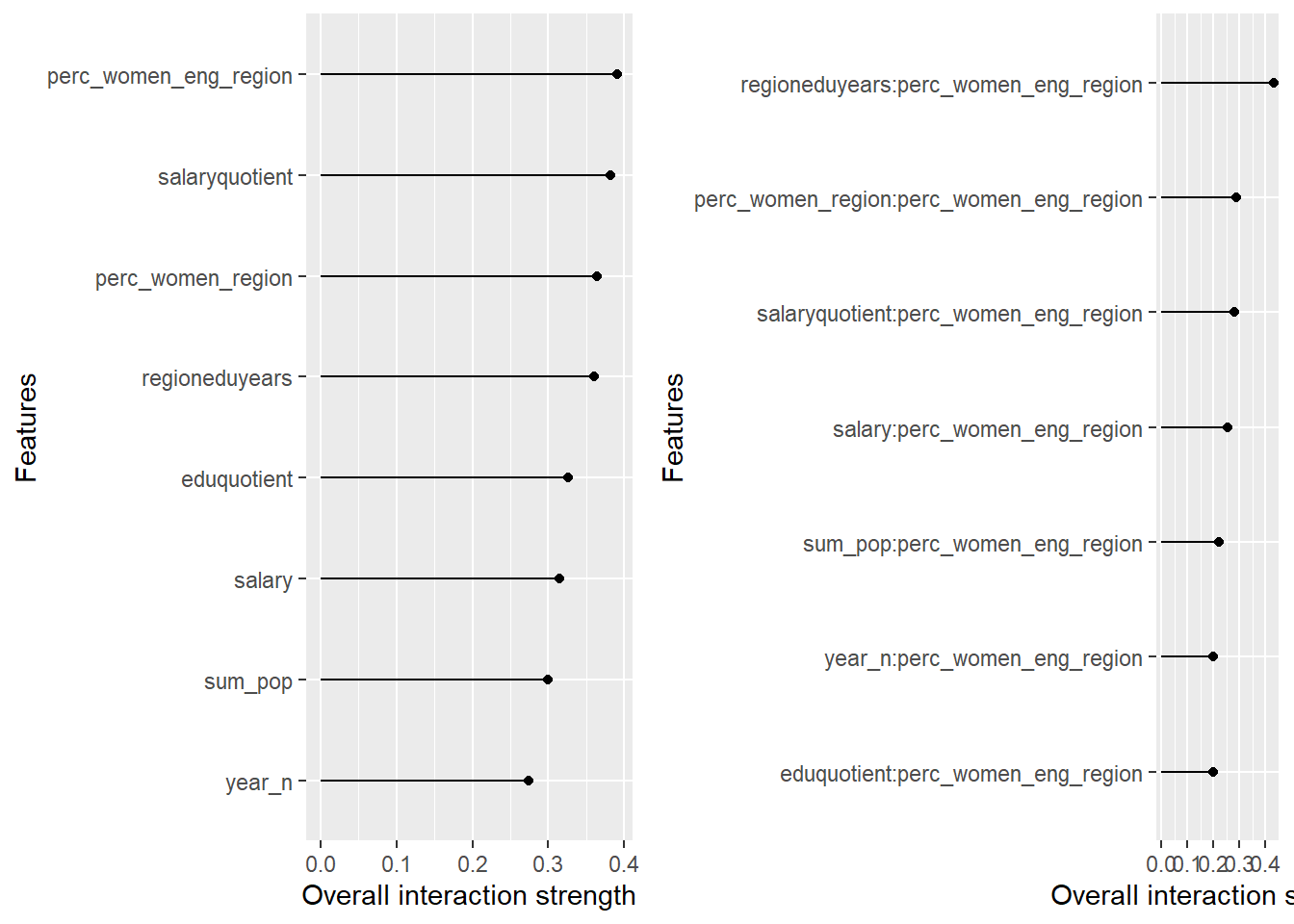Figure 32: , Year 2014 – 2018

```## TableGrob (1 x 2) "arrange": 2 grobs
##   z     cells    name           grob
## 1 1 (1-1,1-1) arrange gtable[layout]
## 2 2 (1-1,2-2) arrange gtable[layout]
##
## Conditional Inference Tree
##
## 38 samples
##  8 predictor
##
## Pre-processing: principal component signal extraction (8), centered (8),
##  scaled (8)
## Resampling: Cross-Validated (10 fold)
## Summary of sample sizes: 34, 34, 35, 34, 34, 34, ...
## Resampling results across tuning parameters:
##
##   mincriterion  RMSE      Rsquared   MAE
##   0.01          4244.251  0.7539372  2824.5
##   0.50          4244.251  0.7539372  2824.5
##   0.99          4244.251  0.7539372  2824.5
##
## RMSE was used to select the optimal model using the smallest value.
## The final value used for the model was mincriterion = 0.99.
##     RMSE Rsquared      MAE
##        0        1        0```

`## Warning: Removed 38 rows containing missing values (geom_point).`Figure 33: , Year 2014 – 2018

```## TableGrob (2 x 2) "arrange": 4 grobs
##   z     cells    name           grob
## 1 1 (1-1,1-1) arrange gtable[layout]
## 2 2 (1-1,2-2) arrange gtable[layout]
## 3 3 (2-2,1-1) arrange gtable[layout]
## 4 4 (2-2,2-2) arrange gtable[layout]```

```## Warning in .subset2(public_bind_env, "initialize")(...): Model error is 0,
## switching from compare='ratio' to compare='difference'```Figure 34: , Year 2014 – 2018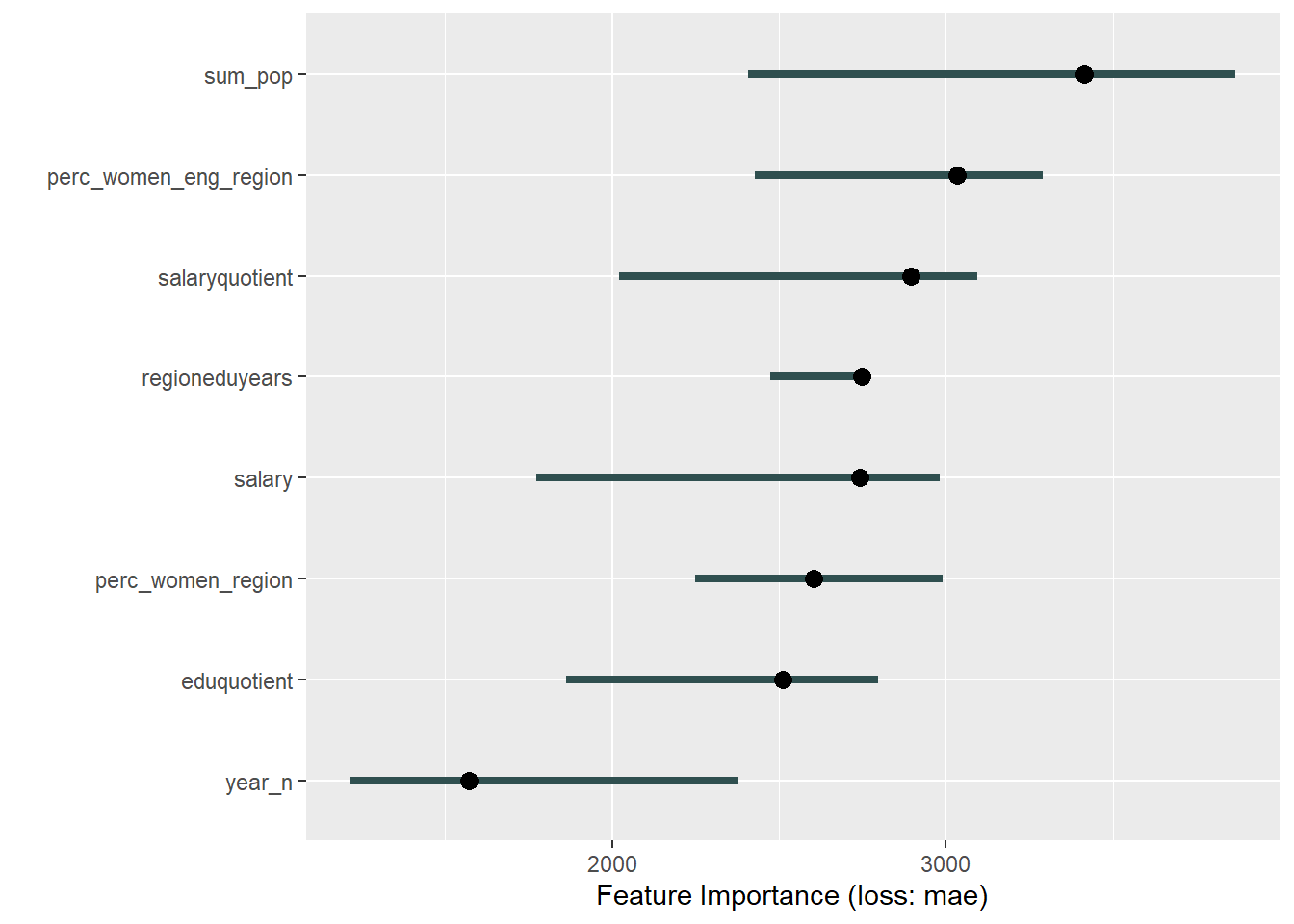Figure 35: , Year 2014 – 2018Figure 36: , Year 2014 – 2018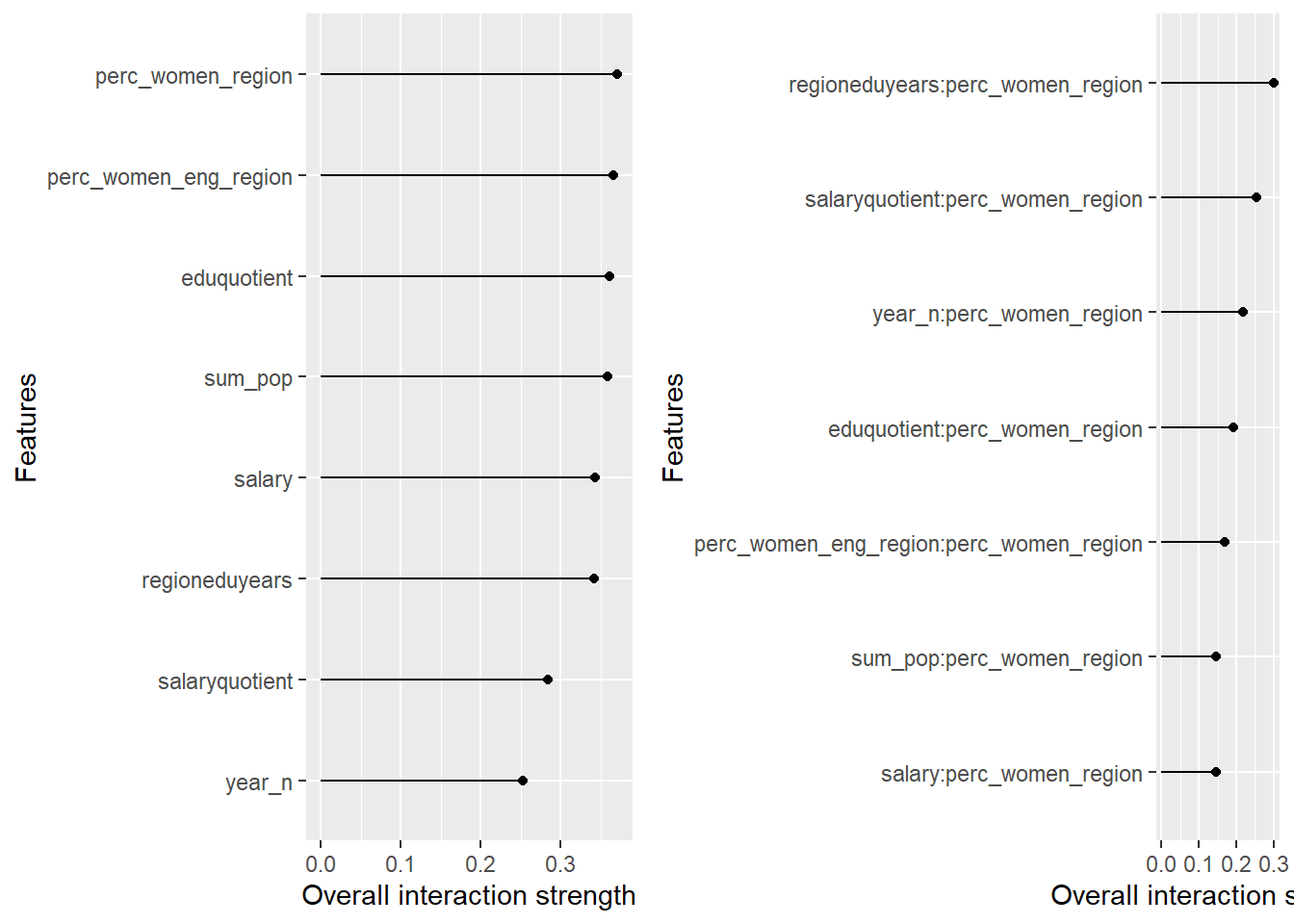Figure 37: , Year 2014 – 2018

```## TableGrob (1 x 2) "arrange": 2 grobs
##   z     cells    name           grob
## 1 1 (1-1,1-1) arrange gtable[layout]
## 2 2 (1-1,2-2) arrange gtable[layout]```